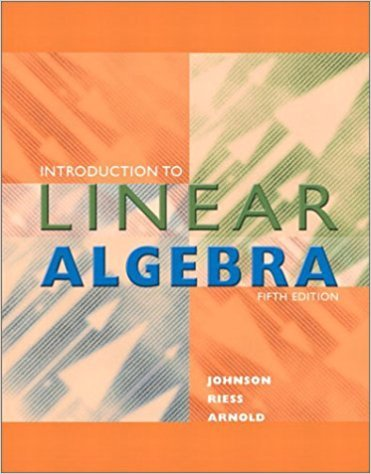×
×

# Solutions for Chapter 1.8: Introduction to Linear Algebra 5th Edition## Full solutions for Introduction to Linear Algebra | 5th Edition

ISBN: 9780201658590Solutions for Chapter 1.8

Solutions for Chapter 1.8
4 5 0 256 Reviews
21
5
##### ISBN: 9780201658590

Since 33 problems in chapter 1.8 have been answered, more than 7146 students have viewed full step-by-step solutions from this chapter. Chapter 1.8 includes 33 full step-by-step solutions. Introduction to Linear Algebra was written by and is associated to the ISBN: 9780201658590. This expansive textbook survival guide covers the following chapters and their solutions. This textbook survival guide was created for the textbook: Introduction to Linear Algebra , edition: 5.

Key Math Terms and definitions covered in this textbook
• Affine transformation

Tv = Av + Vo = linear transformation plus shift.

• Change of basis matrix M.

The old basis vectors v j are combinations L mij Wi of the new basis vectors. The coordinates of CI VI + ... + cnvn = dl wI + ... + dn Wn are related by d = M c. (For n = 2 set VI = mll WI +m21 W2, V2 = m12WI +m22w2.)

• Cholesky factorization

A = CTC = (L.J]))(L.J]))T for positive definite A.

• Column space C (A) =

space of all combinations of the columns of A.

• Complex conjugate

z = a - ib for any complex number z = a + ib. Then zz = Iz12.

• Ellipse (or ellipsoid) x T Ax = 1.

A must be positive definite; the axes of the ellipse are eigenvectors of A, with lengths 1/.JI. (For IIx II = 1 the vectors y = Ax lie on the ellipse IIA-1 yll2 = Y T(AAT)-1 Y = 1 displayed by eigshow; axis lengths ad

• Free columns of A.

Columns without pivots; these are combinations of earlier columns.

• Gauss-Jordan method.

Invert A by row operations on [A I] to reach [I A-I].

• Graph G.

Set of n nodes connected pairwise by m edges. A complete graph has all n(n - 1)/2 edges between nodes. A tree has only n - 1 edges and no closed loops.

• Hermitian matrix A H = AT = A.

Complex analog a j i = aU of a symmetric matrix.

• Jordan form 1 = M- 1 AM.

If A has s independent eigenvectors, its "generalized" eigenvector matrix M gives 1 = diag(lt, ... , 1s). The block his Akh +Nk where Nk has 1 's on diagonall. Each block has one eigenvalue Ak and one eigenvector.

• Linearly dependent VI, ... , Vn.

A combination other than all Ci = 0 gives L Ci Vi = O.

• Minimal polynomial of A.

The lowest degree polynomial with meA) = zero matrix. This is peA) = det(A - AI) if no eigenvalues are repeated; always meA) divides peA).

• Pseudoinverse A+ (Moore-Penrose inverse).

The n by m matrix that "inverts" A from column space back to row space, with N(A+) = N(AT). A+ A and AA+ are the projection matrices onto the row space and column space. Rank(A +) = rank(A).

• Random matrix rand(n) or randn(n).

MATLAB creates a matrix with random entries, uniformly distributed on [0 1] for rand and standard normal distribution for randn.

• Rotation matrix

R = [~ CS ] rotates the plane by () and R- 1 = RT rotates back by -(). Eigenvalues are eiO and e-iO , eigenvectors are (1, ±i). c, s = cos (), sin ().

• Singular matrix A.

A square matrix that has no inverse: det(A) = o.

• Spectrum of A = the set of eigenvalues {A I, ... , An}.

Spectral radius = max of IAi I.

• Unitary matrix UH = U T = U-I.

Orthonormal columns (complex analog of Q).

• Volume of box.

The rows (or the columns) of A generate a box with volume I det(A) I.

×